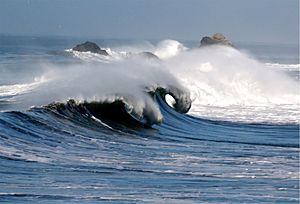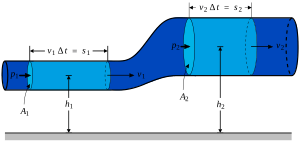# Navier–Stokes equations facts for kids

Kids Encyclopedia FactsSimulating and calculating the dispersion and refraction of water waves is one area where the equations can be used.
MechanicsThe Navier–Stokes equations are mathematical equations that describe the motion of fluids. The equations are named after Claude-Louis Navier and George Gabriel Stokes. The equations result from applying Newton's second law to fluid dynamics with the belief that the fluid stress is the sum of a diffusing viscous term (in relation to the gradient of velocity), plus a pressure term.

They are very useful because they describe the physics of many things of academic and economic interest. They may be used to model the weather, ocean currents, water flow in a pipe, the air's flow around a wing, and motion of stars inside a galaxy. The Navier–Stokes equations in their full and simplified forms help with the design of aircraft and cars, the study of blood flow, the design of power stations, the analysis of pollution, and many other things. Together with Maxwell's equations they can be used to model and study magnetohydrodynamics.

The Navier–Stokes equations are also of great interest in a purely mathematical sense. Somewhat surprisingly, given their wide range of practical uses, mathematicians have not yet proven that in three dimensions solutions always exist (existence), or that if they do exist, then they do not contain any singularity (or infinity or discontinuity) (smoothness). These are called the Navier–Stokes existence and smoothness problems. The Clay Mathematics Institute has called this one of the seven most important open problems in mathematics, and offered a US\$1,000,000 prize (approx. €0.7M or £0.6M as of September 2009) for a solution or a counter-example.

The Navier–Stokes equations dictate not position but rather velocity. A solution of the Navier–Stokes equations is called a velocity field or flow field, which is a description of the velocity of the fluid at a given point in space and time. Once the velocity field is solved for, other quantities of interest (such as flow rate or drag force) may be found. This is different from what one normally sees in classical mechanics, where solutions are typically trajectories of position of a particle or deflection of a continuum. Studying velocity instead of position makes more sense for a fluid, however for visualization purposes one can compute various trajectories.

## Images for kidsNavier–Stokes equations Facts for Kids. Kiddle Encyclopedia.